# Komlos conjecture, Gaussian correlation conjecture, and a bit of machine learning

Today I would like to talk (somewhat indirectly) about a beautiful COLT 2014 paper by Nick Harvey and Samira Samadi. The problem studied in this paper goes as follows: imagine that you have a bunch of data points in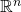with a certain mean value. Your goal is to generate a sequence out these data points, such that the partial averages of this new sequence are as close as possible to. The “herding” procedure of Max Welling has the property that the average of the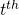first terms in the new sequence is at distance at mostfrom(this was shown by Francis Bach, Simon Lacoste-Julien and Guillaume Obozinski by viewing the herding procedure as a simple Frank-Wolfe). In their paper Nick and Samira improves the dependency on the dimension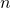in the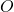notation by using a new algorithm inspired by the theory of discrepancy. In the rest of this post I would like to discuss the basic notions of this theory. What follows is a transcription of what Ronen Eldan (a postdoc in the theory group at MSR) explained to me (of course all errors are mine). I will use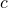and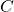to denote absolute numerical constants that take whatever value I need them to take.

Discrepancy theory

Consider a large finite set of vertices, and a size-setof subsets of vertices (in other wordsis an hypergraph). For sake of simplicity we will assume here that elements ofare also of size. We are now interested in the following combinatorial optimization problem: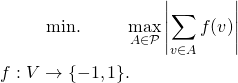In words, one is looking for a partitioningof the vertices, so as to have the hyperedgesas balanced as possible.

Alright, so let’s try something simple, just randomly partition the vertices: clearly a simple union bounds shows that the obtained objective value in this case is of order. Denote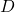(for Discrepancy) the smallest objective value (in particular we just saw that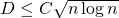). A beautiful result of Joel Spencer shows that one can always do much better than random partitioning:

Theorem (Spencer’s six deviations, 1985):.

We will now see a splendid (partial) proof of this result. Letbe the Gaussian measure in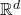(in the following we will take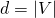). First let me remind you of a basic fact about this measure, and a related conjecture. A set of the form, for some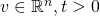, is called a slab.

Theorem (Sidak, 1967): Let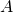be a symmetric convex set andbe a slab, then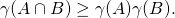Obviously there is equality ifandare two orthogonal slabs, and the theorem says (in particular) that an angle between the slabs would imply positive correlation.

Conjecture (Gaussian correlation): Letandbe two symmetric convex sets, thenOk, now back to the proof of Spencer’s theorem. First, let’s rewrite things suggestively in terms of the slabs,. We want to show that there exists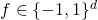such that. Using Sidak’s theorem one clearly has(withbeing roughly like). From there it is now a fairly short route to show that, if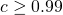(say), then the intersection ofwith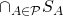must be non-empty. Ronen showed me a non-trivial (and algorithmically non-efficient) 5 lines argument for this but I think that it’s yet unpublished work so I won’t reproduce it here. Another proof of this, which yields an efficient algorithm for finding a point in the intersection, is given in this very nice paper of Lovett and Meka.

Extensions

In what we discussed so far the underlying hypergraph was completely arbitrary. For some specific graphs one can clearly improve the bound on. Indeed if all hyperedges are disjoint, then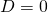. More interestingly, Beck and Fiala showed in 1981 that, if each vertex appears in at mosthyperedges, then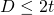. They also conjectured that the correct bound should be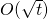. In 1998 Banaszczyk showed a bound ofusing the following theorem (which is a variant of the result mentioned above):

Theorem (Banaszczyk, 1998): Letbe a convex set such that, and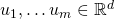such that. Then there existssuch that.

The longstanding Komlos’ conjecture says that in fact this holds for(despite the fact that the Gaussian measure of this set is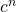).

Finally let me mention another open problem, which is the matrix version of Spencer’s six deviations, see this blog post by Raghu Meka for details.

This entry was posted in Optimization, Probability theory, Theoretical Computer Science. Bookmark the permalink.

## 5 Responses to "Komlos conjecture, Gaussian correlation conjecture, and a bit of machine learning"

•••••